Restrictions on Constitutive Relations

# Restrictions on Constitutive Relations Notes - Civil Engineering (CE)

## Document Description: Restrictions on Constitutive Relations for Civil Engineering (CE) 2022 is part of Civil Engineering (CE) preparation. The notes and questions for Restrictions on Constitutive Relations have been prepared according to the Civil Engineering (CE) exam syllabus. Information about Restrictions on Constitutive Relations covers topics like and Restrictions on Constitutive Relations Example, for Civil Engineering (CE) 2022 Exam. Find important definitions, questions, notes, meanings, examples, exercises and tests below for Restrictions on Constitutive Relations.

Introduction of Restrictions on Constitutive Relations in English is available as part of our Civil Engineering (CE) preparation & Restrictions on Constitutive Relations in Hindi for Civil Engineering (CE) courses. Download more important topics, notes, lectures and mock test series for Civil Engineering (CE) Exam by signing up for free. Civil Engineering (CE): Restrictions on Constitutive Relations Notes - Civil Engineering (CE)
 1 Crore+ students have signed up on EduRev. Have you?

Restrictions on constitutive relation
There are certain restrictions, guidelines and principles that are common which the constitutive relations for different materials and under different conditions of interest, should satisfy. Thus, we require the constitutive relation to ensure that the value of the state variables for a given state of the body are independent of the placement of the body in the Euclidean space and to honor the coordinate transformation rules. There is also, the concept of material symmetry which to a large extent determines the variables in the constitutive relation. The following section is devoted towards understanding these concepts.

Restrictions due to objectivity
While the physical body was given a mathematical representation, we made two arbitrary choices regarding:
1. The region in the Euclidean point space to which the body is mapped.
2. The orientation of the basis vectors with respect to which the position, velocity, acceleration, etc. of the material particles are defined.
Since these choices are arbitrary, the state of the body cannot depend on these choices. (Clearly, a body subjected to a particular load cannot fail just because, the body is mapped on to a different region of Euclidean point space or a different set of basis vectors is used.) Hence, the constitutive relations that relate these state variables with the independent variables, i.e., kinematic variables, should also not depend to these choices.
The value of state variables variables have to be invariant with respect to the above choices because they mathematically describe the state of the body. We emphasize that the value of the state variables have to be invariant and not same. Thus, while the value of scalar valued state variables like density have to be the same, the components of tensor valued state variables like stress will change with the choice of the basis according to the transformation rules discussed in section 2.6. However, as pointed out in 2.6 just because the components of a tensor is different does not mean they are different tensors. Only, if the value of at least one of the certain scalar valued functions of the components of the tensor are different5 , the two tensors are said to be different.

Restriction due to non-uniqueness of placers
In this section, we shall explore the restrictions that needs to be placed on the constitutive relations so that they remain invariant for the different equivalent mappings of the body on to the Euclidean point space. From a physical standpoint, the same restriction could be viewed as requiring the constitutive relation of the body be the same irrespective of where the body is. That is, say, the deflection of the beam to a given set of loads should remain the same whether it is tested in Chennai or Delhi or Washington or London.
It should be realized that in the different mappings of the body onto the Euclidean space, it is the point space that changes and not the vector space. If the vector space were to change, it means that we are changing the basis vectors. Further, it is assumed that the scale used to measure distances between two points does not change. Consequently, the distance between any two points in the body does not change due to these different mappings of the body. Hence, this restriction, as we shall see, tantamount to requiring that the state variables be invariant to superposed rigid body rotation of the current or the reference configuration.

Let x and x+ denote the position vector of a material particle at time t, mapped on to different regions of the Euclidean point space. Then, they are

 5For example, if A is a second order tensor then one of the following: tr(A), tr(A−1 ), det(A), must be different. There is nothing unique about the choice of the scalar valued functions of A. One could have chosen tr(A2 ) instead of det(A) or more generally, tr(Am) where m takes some integer value.related by the equation:

x+ = Qx + c,                                  (6.5)

where Q and c are functions of time, t and Q is an orthogonal tensor, not necessarily a proper orthogonal tensor6 . While it is easy to see that (6.5) preserves the distance between any two points, the converse can be proved by following the same steps as that outlined in section 3.10.1.

Since, similar non-uniqueness exist with respect to placers for the reference configuration

X+ = QoX + co,                                (6.6)

where X and Xare the position vectors of same material particle mapped on to the different regions of the Euclidean point space, Qo is a constant orthogonal tensor and co is a constant vector. Next, we like to compute the standard kinematical quantities and see how they change due to these equivalent mappings of the body on to the Euclidean point space. This is a prerequisite to find the restrictions on the constitutive relations due to this requirement. We begin by considering the transformations of the motion field,

χ. Let x = χ(X, t), x+ = χ +(X+, t)                                              (6.7)

then using equations (6.5) and (6.6) we obtain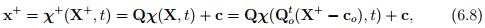Now, we can find the relation between the deformation gradients, F and F+ defined as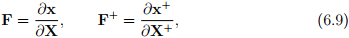using (6.6), (6.8) and chain rule for differentiation to be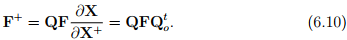Next, we find the relation between the velocity fields, v and v+ defined as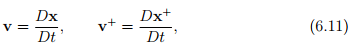6We allow improper orthogonal tensors because what is considered as top or bottom, left or right is subjective.

using equation (6.5) as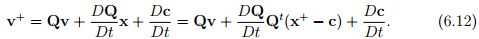Similarly, we obtain the relationship between accelerations a and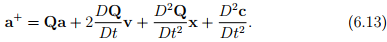In order to simplify equations (6.12) and (6.13) we introduce, the skew tensor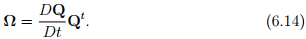To show that is a skew tensor, taking the total time derivative of the relation QQt = 1, we obtain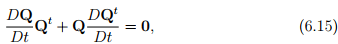from which the required result follows. In lieu of definition (6.14), equations (6.12) and (6.13) can be written as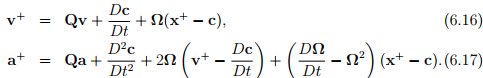where we have made use of the identity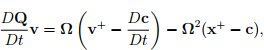to obtain (6.17).
Now, we would like to see how the displacement transforms due to different placements of the body in the Euclidean point space. Recalling that the displacement field is defined as:

u = x − X, u+ = x+ − X+.                                                 (6.18)

The Eulerian displacement gradient transforms like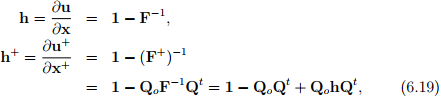where we have used (6.10) and (3.34). Similarly, the Lagrangian displacement gradient, is computed to be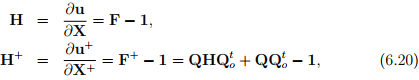using (6.10) and (3.33). It is apparent from the above equations that displacement gradient transforms as QhQt (or QoHQtoonly if Q = Qo. Then, it is straightforward to show that the right and left Cauchy-Green deformation tensor transform as:

C+ = QoCQto ,                                B+ = QBQt .                                (6.21)

Next, we examine what happens to stress tensors due to different placements of the body in the current configuration. Recalling traction is related to the Cauchy stress, by the relation t(n) = σn, where n is the outward unit normal at a point x on the boundary surface ∂Bt of an arbitrary region Bt . As evidenced from the figure (6.2) a different placer of the body in the Euclidean space, in general, alters the orientation of the normal with respect to a fixed set of basis vectors. Let n and n+ denote the outward unit normals to the same material surface ∂B and at the same material particle, P, in two different placements of the body in the Euclidean space, Bt and B+t . Since, we are interested in the same material surface, we can appeal to Nanson’s formula (3.72) to relate the unit normals as

n+ = Qn.                                (6.22)

where we have made use of the equation (6.5) which provides the relationship between the placements Bt and B+t .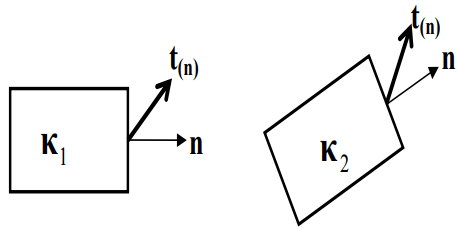Figure 6.2: Two placements (κ1, κ2) of the same body in the same state along with a unit normal (n) and traction (t(n)).

Similarly, the traction vector t(n) and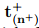acting on the same material surface, ∂B and at the same material particle P, in two different placements of the body, Bt and B+t are related through:= Qt(n) .                            (6.23)

To obtain the above equation we have made use of the fact that the normal and shear traction acting on a material surface in two different placements of the body in the current configuration has to be same, i.e.,� n+ = t(n)n. Then, (6.23) is obtained by recognizing that (Qt− t(n)) � n = 0 for any choice of n where we have made use of (6.22). Since,= σ+n+ and t(n) = σn, we obtain

Qσn = σ+Qn,                                (6.24)

using equations (6.22) and (6.23) and the above equation has to hold for any choice of n. Therefore, the Cauchy stress will transform as

σ+ = QσQt .                              (6.25)

Since, the definition of Cauchy stress is independent of the choice of reference configuration, different placers of the reference configuration, does not cause any change in the value of the Cauchy stress.

Next, let us see how body forces transform. These forces are essentially action at a distance forces acting along a straight line joining the material particles in the body under investigation to another point in space belonging to some other body. Thus, if r is the position vector of the point belonging to another body, then the direction along which b acts, is given by (x−r)/(| x−r |). Then, in a different placement of the body the body force acts along a direction (x+ − r)/(| x+ − r+ |). Because the new placement of the body is related to its original placement by equation (6.5), we obtain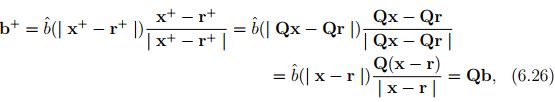where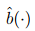denotes a function of the distance between two points of interest. Here r+ = Qr + c because the transformation rule is applicable not only to points that the body under study occupies but to its neighboring bodies as well. This is required so that the relationship between the body under investigation and its neighbors are preserved. Finally, for future reference, div(σ) transforms due to equivalent placements of the body as,

div++) = div(σ+)Q = div(QσQt )Q = Qdiv(σ),                                        (6.27)

where div+ denotes the divergence with respect to x+.

Till now, we have just seen how various quantities transform between different placements of the same body in the same state. Using this we are in a position to state what restriction the requirement that the state variables be invariant to the different placers of the body in the same state places on the constitutive relation, given it depends on certain kinematic quantities and other state variables. For example, as we saw above for elastic process the stress is a function of deformation gradient, i.e.,

σ = f(F).                                         (6.28)

Now, due to different placement of the body in the reference and current configurations,

σ+ = f(F+).                         (6.29)

Note that the function does not change and this is what restricts the nature of the function. Using equations (6.25) and (6.10), (6.29) can be written as

σ+ = QσQt = f(F+) = f(QFQto ).                 (6.30)

Substituting for σ from equation (6.28) we obtain a restriction of the form of the function f as:

Qf(F)Qt = f(QFQto ),                   (6.31)

for any orthogonal tensors Q and Qo and F ∈ D ⊆ Lin+, the set of all linear transformations whose determinant is positive.

The question on how to obtain the form of the function, given the restriction (6.31), is dealt in detail next. To understand how this restriction (6.31) fixes the form of the tensor-valued tensor function, let us look at a similar requirement on scalar-valued scalar function, g(x). Say the restriction on this scalar function is: g(m ∗ x) = m ∗ g(x), where m is some arbitrary constant. It then immediately tells that the function is a linear function, i.e., g(x) = a ∗ x, where a is some constant. Similarly, it transpires that the restriction (6.31) requires,

σ = f(F) = α01 + α1B + α2B−1 ,                           (6.32)

where αi = �i(J1, J2, J3),

J1 = tr(B), J2 = tr(B−1 ), J3 = [det(B)]1/2 = det(F),                 (6.33)

are the invariants of B.

Now, we show how equation (6.32) is obtained from the restriction (6.31). Towards this, first we set Q = 1 to obtain,

f(F) = f(FQto ) = f(VR) = f(VRQto ),                          (6.34)

where we have made use of the polar decomposition theorem (2.116). Then, let us pick Qo = R, since R is also an orthogonal tensor. With this choice equation (6.34) yields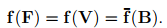(6.35)

The last equality arises because B = V2 and square-root theorem, (2.147) ensures the existence of an unique V such that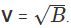Next, we shall again appeal to equation (6.31) but now we shall not set Q to be 1 to obtain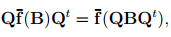(6.36)

Q ∈ O. The above equation can be rewritten as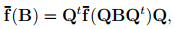(6.37)

Q ∈ O.
Theorem 7.1: A symmetric second order tensor valued function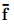defined over the space of symmetric second order tensors, satisfies (6.37) if and only if it has a representation

σ =(B) = α01 + α1B + α2B2                     (6.38)

where α0, α1, α2 are functions of principal invariants of B, Ii , i.e.,

αi =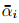i(I1, I2, I3).                                 (6.39)

Proof: 7 Before proving the above theorem we prove the following theorem:

Theorem 7.2: Let α be a scalar function defined over the space of symmetric positive definite second order tensors. Then α(QBQt ) = α(B) ∀ Q ∈ O if and only if there exist a function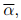, defined on R+ �R+ �R+, such that α(B) =1, λ2, λ3), where1, λ2, λ3) is insensitive to permutations of λi and λ1, λ2, λ3 are the eigenvalues of B. Hence, α(B) = �(I1, I2, I3). Proof: 8 Writing B in the spectral form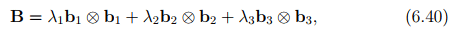where bi ’s are the ortho-normal eigenvectors of B and hence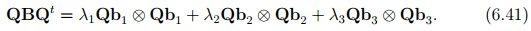Since, α(QBQt ) = α(B) ∀ Q ∈ O, α(B) must be independent of the orientation of the principal directions of B and must depend on B only through its eigenvalues, λ1, λ2, λ3. Next, choose Q to be a rotation of π/2 about b3 so that Qb1 = b2, Qb= −b1 and Qb= b3. Hence,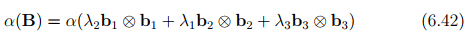7Adapted from Serrin  and Ogden . The original proof is due to Rivlin and Ericksen
 8Adapted from Ogden 

from which we deduce that1, λ2, λ3), =1, λ2, λ3),. In similar fashion it can be shown that α is insensitive to other permutations of λi ’s.

Finally, recalling that the eigenvalues are the solutions of the characteristic equation

λ3 − I1λ2 + I2λ − I3 = 0,                                                                               (6.43)

in principal, λi ’s can be expressed uniquely in terms of the principal invariants and hence α(B) = �(I1, I2, I3).
The converse of the theorem 7.2 is proved easily from the property of trace and determinants. Hence, we have proved theorem 7.2.

Theorem 7.3: If f satisfies (6.37) then the eigenvalues of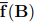are functions of the principal invariants of B.
Proof: Let γ(B) be the eigenvalue of. Then,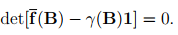(6.44)

The corresponding eigenvalue of(QBQt ) is γ(QBQt ) and hence

det[(QBQt ) − γ(QBQt ) 1 ] = 0.                                              (6.45)

This can be written as

det[Q{(B) − γ(QBQt )1}Qt ] = 0,                                              (6.46)

by using (6.37) and the relation QQt = 1. Using the property of determinants the above equation reduces to

det[(B) − γ(QBQ)1] = 0,                                                       (6.47)

which has to hold for all Q ∈ O. Comparing (6.44) and (6.47)

γ(QBQt ) = γ(B),                                                                          (6.48)

for all Q ∈ O, which by theorem 2.2 implies that γ(B) = �(I1, I2, I3).

Theorem 7.4: If σ =(B) satisfies (6.37) thenis coaxial with B, i.e., the principal directions of B andwould be the same. Proof: Consider an eigenvector b1 of B and define an orthogonal transformation Q by

Qb1 = −b1, Qbj = bj          if        b1bj = 0,                                (6.49)

i.e. Q is a reflection on the plane normal to b1. Now, QBQt = B and hence, by (6.37), Qσ = σQ. We therefore have

Q(σb1) = σ(Qb1 ) = −σb1,                                                           (6.50)

and we see that Q transforms the vector σb1 into its opposite. Since, the only vectors transformed by the reflection Q into their opposites are the multiples of b1, it follows that b1 is an eigenvector of σ. Similarly, it can be shown that every eigenvector of B is also an eigenvector of σ. Hence,is coaxial with B.
Now we prove theorem 7.1.
Clearly, if (6.38) along with (6.39) holds then (6.37) is satisfied and hence we have to prove only the converse.
It follows from theorem 7.3 and theorem 7.4 thatis coaxial with B and its eigenvalues are functions of the principal invariants of B. Let λ1, λ2, λ3 and f1,f2,fbe the eigenvalues of B and f(B) respectively and consider the equations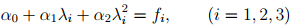(6.51)

for the three unknowns α0, α1, α2. Assuming the λi and fi are given and that λi ’s are distinct it follows that αi ’s are determined uniquely in terms of λi and fwhich are themselves determined uniquely by the principal invariants of B. Thus, since B is coaxial with) and αi are functions of the principal invariants of B; equation (6.38) follows from (6.51) provided the eigenvalues of B are distinct, of course. When the eigenvalues of B are not distinct α2 or α1 and α2 could be chosen arbitrarily, depending on whether the algebraic multiplicity of the eigenvalues is 2 or 3 respectively. However, this choice may cause some αi to become discontinuous even whenremains continuous, Truesdell and Noll  and Serrin  provide example of such cases. Finally, from Cayley-Hamilton theorem, (2.142) we obtain

B2 = I1B − I21 + I3B−1 .                                                         (6.52)

Then, observing that the principal invariants of the positive definite, B, are related bijectively to the invariants J1, J2 and J3, as defined in (6.33), we note that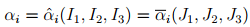(6.53)

for i = (0, 1, 2). Substituting (6.52) and (6.53) in (6.38) we obtain

σ = α01 + α1B + α2B−1 .                                                     (6.54)

Before concluding this section let us investigate a couple of issues. The first issue is whether the balance laws should also be invariant to different placements of the body in the same state. A straight forward calculation will show that balance of mass is invariant to different placements of the same body in the same state. However, balance of linear momentum and hence angular momentum are not. In fact, due to equivalent placements of the body, the balance of linear momentum equation transforms asBut for the above equation to be consistent, it is required that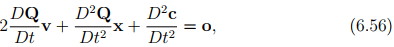since, div(σ) + ρb = ρa. The above equation holds only for placements for which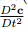= o and Q(t) = Qc , a constant. Thus, a school of thought requires the constitutive relations describing the state variables to be invariant only for placements of the body related as:

x+ = Qcx + ctt + co,                                                                   (6.57)

where Qc is a constant orthogonal tensor, ct and co are constant vectors. Equation (6.57) is called Galilean transformation.
The other school of thought, argues that equivalent placements of the body in a particular state is a thought experiment to obtain some restriction on the constitutive relations describing the state variables which is not applicable for balance laws. The rational behind their argument is that for a given body force, the balance laws will hold only for certain motions of the body9 . On the other hand, there are no such restrictions for the constitutive relations. Hence, there is no inconsistency if motions that are not admissible according to the balance law are used to find restrictions on the constitutive relations. We find merit in this school of thought.
The second issue is on the existence of unique placer for the reference configuration. Some researchers are of the opinion that the placer to the reference

 9As we shall see, within the realms of finite elasticity, Ericksen  has showed that in the absence of body forces, deformations of the form: r = r(R), θ = Θ, z = Z, where (R, Θ, Z) and (r, θ, z) are the coordinates of a material particle in the reference and current configuration respectively, is not possible in some compressible materials.

configuration has to be unique for agreement on the material symmetry of the body. In section 6.3.2, we show that the opinion of these researchers is incorrect.

Restrictions due to non-uniqueness of the basis vectors
We have been writing equations in direct form, that is independent of the choice of basis vectors. To beginners it is natural to ask the question, if we were to continue to write equations in direct form what restriction can this choice of basis vectors place? To answer this question let us look at an example. Say, some person investigating the response of some body subjected to some process finds that Cauchy stress, σ is related to the deformation gradient, F, as σ = α(F − 1), where α is a constant. Even though we have written in direct notation, say, we change the basis vectors used to describe the reference configuration, then the matrix components of the Cauchy stress will not change. However, the matrix components of the deformation gradient will change resulting in a contradiction and inadmissability of the proposed relation.

To elaborate, recall that σ = σije⊗ eand F = Fijei ⊗ Ej , where ei is the basis vectors used to represent the current configuration and Ej is the basis vectors used in the reference configuration. Then, let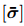and [] represent the matrix components of the Cauchy stress and deformation gradient with respect to the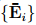basis. Similarly, let [σ] and [F] denote the matrix components of the Cauchy stress and deformation gradient with respect to the {Ei} basis. It follows from the transformation laws 2.6 that= [σ], [] = [F][Q],                        (6.58)

where Qij = Ei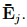Immediately, the contradiction in the constitutive relation, σ = α(F − 1) is apparent. Let us see what restriction is placed on the form of the function (or functional) to ensure that it obeys the necessary transformation rules. Thus, say, we postulate that: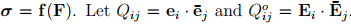Due to this transformation of the basis vectors in the current and the reference configuration, the stress transforms as= [Q]t [σ][Q] and the deformation gradient transforms as [] = [Q]t [F][Qo ]. Then, we require that= [f([])]. Hence, the function f(�) should be such that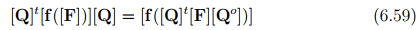for any [Q] and [Qo ] such that [Q][Q]t = [Q]t [Q] = [Q][Qo ]t = [Q]t [Qo ] =  and for all F ∈ D ⊆ Lin+. While it is non-trivial to deduce the form of the function f(F) satisfying the condition (6.59), it is easy to verify that

f(F) = α0+ α1B + α2B−1 ,                                                            (6.60)

where B = FFt , αi =�i(tr(B), tr(B−1 ), det(F)) upholds the condition (6.59). Looking at restriction (6.31) and (6.59) one might wonder on the need for (6.59). Because if (6.31) were to hold then (6.59) will always hold. However, recognize that (6.59) is a different requirement than (6.31) and these differences would be apparent for cases where the constitutive relation depends on velocity or its derivatives. To see this, a change of basis used to describe the current configuration results in the velocity vector transforming as [Q][v], which is different from (6.16) and hence the difference between the restrictions (6.59) and (6.31). To clarify, even if the basis were to change continuously with time, the velocity will transform as [Q(t)][v] because the choice of the basis determines only the components of the velocity vector and not the velocity of the particle which depends only on the relative motion of the particle and the observer.

Restrictions due to Material Symmetry
Before looking at the concept of material symmetry, we have to understand what we mean by the body being homogeneous or inhomogeneous. Intuitively, we think that a body is homogeneous if it is made up of the same material. However, there is no particular variable in our formulation that uniquely characterizes the material and only the material. But the constitutive relation (or equation of state) which relates the state variables and the kinematic variable depends on the material. However, since the value of the kinematic variable, say the displacement or the deformation gradient, depends on the configuration used as reference, the constitutive relation also depends on the reference configuration or more particularly on the value of the state variables in the configuration used as reference. Hence, just because the constitutive relation for two particles are different does not mean that they are different materials, the difference can arise due to the use of different configurations being used as reference.

Consequently, mathematically, we say that two particles in a body belong to the same material, if there exist a configuration in which the density and
temperature of these particles are same and with respect to which the constitutive equations are also same. In other words, what we are looking at is if the value of the state variables evolve in the same manner when two particles along with their neighborhood are subjected to identical motion fields from some reference configuration in which the value of the state variables are the same.

A body that is made up of particles that belong to the same material is called homogeneous. If a body is not homogeneous it is inhomogeneous. Now, say we have a body, in which different subsets of the body have the same constitutive relation only when different configurations are used as reference, i.e., any configuration that the body can take without breaking its integrity would result in different constitutive relations for different material particles, then the issue is how to classify such a body. Any body with residual stresses10 like shrink fitted shafts, biological bodies are a couple of examples of bodies that fall in this category. One school of thought is to classify these bodies also as inhomogeneous, we subscribe to this definition simply for mathematical convenience.

Having seen what a homogeneous body is we are now in a position to understand what an isotropic material is. Consider an experimentalist who has mathematically represented the reference configuration of a homogeneous body, i.e., identify the region of the Euclidean point space that this body occupies, has found the spatial variation of the state variables. Now, say without the knowledge of the experimentalist, this reference configuration of the body is deformed (or rotated). Then, the question is will this deformation (or rotation) be recognized by the experimentalist? Theoretically, if the experimentalist cannot identify the deformation (or rotation), then the functional form of the constitutive relations should be the same for this deformed and initial reference configuration. This set of indistinguishable deformation or rotation forms a group called the symmetry group and it depends on the material as well as the configuration that it is in. If the symmetry group contains all the elements in the orthogonal group11 then the material in that configuration is said to possess isotropic material symmetry. If the symmetry group does not contain all the elements in the orthogonal group, the material in that configuration is said to be anisotropic. There are various classes of

 10The non-uniform stresses field in a body free of boundary traction is called residual stress.11The set of all linear transformations, Q, such that QQt = QtQ = 1 form a group called the orthogonal group.

anisotropy like transversely isotropic, orthorhombic, etc., depending on the elements contained in the symmetry group. Here we like to emphasize on some subtleties. Firstly, we emphasize that the symmetry group of a material depends on the configuration in which it is assessed. Thus, the material in a stress free configuration could be isotropic but the same material in uniaxially stressed state will not be isotropic. Secondly, we allow the body to be deformed because it has been shown  that certain deformations superposed on an uniaxially extended body does not alter the state of the body. Hence, it should be recognized that the symmetry group, G ⊆ H, the unimodular group12, since the volume cannot change in an equivalent placement of the body. In fact, for a perfect gas the symmetry group, G = H. Thirdly, unlike in the restriction due to objectivity, in this case only the body is rotated or deformed virtually, not its surroundings. Even though like in the restriction due to objectivity the rotation or deformation is virtual, it has to maintain the integrity of the body and satisfy the balance laws, i.e., be statically admissible because the rotated or deformed configuration is also in equilibrium.

Now, let us mathematically investigate the restriction material symmetry imposes on the constitutive relation. Continuing with our example, σ = f(F) we examine the restriction on f(F) due to material symmetry. For any G ∈ G, the restriction due to material symmetry requires that

σ = f(F) = f(FG),                   (6.61)

F ∈ D. Of course, for this case the restriction is similar to that obtained for objectivity (6.31), except that now, Q = 1 and Qo is not necessarily limited to orthogonal tensors. However, for isotropic material the symmetry group is the set of all orthogonal tensors only.

To further elucidate the difference between the restriction due to objectivity and material symmetry, consider a material whose response is different along a direction M identified in the reference configuration. Then, σ = f(F,M). Now due to objectivity we require

Qf(F,M)Qt = f(QFQto , QoM),                 (6.62)

for any orthogonal tensors Q and Qo and for any F ∈ D. Due to material symmetry we require

f(F,M) = f(FG,M),                        (6.63)

 12The set of all linear transformations such that their determinant is positive and equal to one, form a group called the unimodular group.

F ∈ D and G ∈ G. In (6.63) the right hand side is not GM because, the rotation (or deformation) G, of the reference configuration is not distinguishable. In other words, G belongs to G only if GM = M. Thus, it immediately transpires that restriction due to material symmetry (6.63) is not same as that due to objectivity (6.62).

It is also instructive to note that if GM = M holds for any an orthogonal tensor, G, then M = o. Thus, the result that for isotropic materials the response would be same in all directions and consequently there are no preferred directions. If the material response along one direction is different, then it is called as transversely isotropic and if its response along three directions are different, it is called orthotropic.

The document Restrictions on Constitutive Relations Notes - Civil Engineering (CE) is a part of Civil Engineering (CE) category.
All you need of Civil Engineering (CE) at this link: Civil Engineering (CE)
 Use Code STAYHOME200 and get INR 200 additional OFF

Track your progress, build streaks, highlight & save important lessons and more!

,

,

,

,

,

,

,

,

,

,

,

,

,

,

,

,

,

,

,

,

,

;